# elements selection problem with DIY Switching Regulator (Buck Converter)

#### exynos

Joined May 21, 2023
5
Hi everyone.

I'm high school student and came here to ask a question to experts.
I'm now designing a simple DC to DC converter (Buck Converter) like this circuit.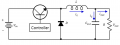But I don't know much so I have proplem with what elements I have to use.
How many Farad value of capacitor and Henry of inductor should I use?

P.S I will make a circuit that is switched according to the charge rate of the capacitor.
If you know, I want to know a MOSFET that allows me to switch to the Hz I want.

#### Ian0

Joined Aug 7, 2020
8,386
It depends on the input voltage, the output voltage, the current and the switching frequency.
If you read the datasheet for the controller you are going to use, it should tell you how to calculate it.

#### exynos

Joined May 21, 2023
5
It depends on the input voltage, the output voltage, the current and the switching frequency.
If you read the datasheet for the controller you are going to use, it should tell you how to calculate it.
There is a controller in the picture I presented, but no controller actually uses it because I intend to connect the base of MOSFET to the capacitor

#### BobTPH

Joined Jun 5, 2013
7,540
There is a controller in the picture I presented, but no controller actually uses it because I intend to connect the base of MOSFET to the capacitor
1. There is no MOSFET in your schematic.

2. If there was, connecting the gate to the capacitor would mot create an oscillator.

I would suggest you use a p-channel MOSFET as the switch, and a CMOS ‘555 as the controller with adjustable duty cycle.

Also, choose an easy frequency to implement, say 100KHz.

Then calculate an inductor based on the ripple current you want and a capacitor capable of smoothing that out.

#### Papabravo

Joined Feb 24, 2006
20,384
Without going into the weeds on the math, the minimum inductor value to ensure continuous conduction is given by: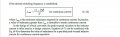D is the nominal duty cycle "on time" for the switch (MOSFET), expressed as a number D, where 0 < D < 1. Small f is the switching frequency in Hertz, and R is the load resistance.

The choice of an output capacitor is chosen according to the maximum acceptable output ripple.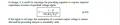As above D is the nominal duty cycle, f is the switching frequency, L is the inductance and the denominator term in parentheses,
$$\Delta V_o/V_o\;\;\;\text{is the output voltage ripple.}$$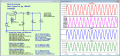Last edited:

#### exynos

Joined May 21, 2023
5
1. There is no MOSFET in your schematic
2. If there was, connecting the gate to the capacitor would mot create an oscillator.
Thank you for replySorry, I'm going to use MOSFET instead of a bipolar transistor.
I have seen the following flicker circuit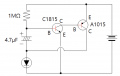And instead of the controller, I thought I could use a similar circuit with the MOSFET's base and capacitor connected.
I wanted the circuit to be switched according to the threshold voltage. (If possible, I think the frequency is determined by the threshold voltage)
By any chance, Is it really impossible to build a circuit like that?

#### Papabravo

Joined Feb 24, 2006
20,384
Thank you for replySorry, I'm going to use MOSFET instead of a bipolar transistor.
I have seen the following flicker circuit
View attachment 294705
And instead of the controller, I thought I could use a similar circuit with the MOSFET's base and capacitor connected.
I wanted the circuit to be switched according to the threshold voltage. (If possible, I think the frequency is determined by the threshold voltage)
By any chance, Is it really impossible to build a circuit like that?
If you intend to use a MOSFET, then do it. Don't just talk about it. You must learn to communicate your ideas precisely. You can't go throwing up any old schematic and say it stands for something else. It marks you as somebody who is not serious about doing things the right way.